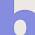# C Programming - Aptitude Questions

1. What values are printed out by the following C program?
```#include <stdio.h> int fun(int x, int y) { x = 2*x + y; return x; } int main(void) { int x = 2, y = 5; y = fun(y, x); x = fun(y, x); printf("%d %d\n", x, y); return 0; } ```

2. What does the following declaration mean?
int (*ptr);
A. ptr is array of pointers to 10 integers
B. ptr is a pointer to an array of 10 integers
C. ptr is an array of 10 integers
D. ptr is an pointer to array

3. Is there any difference int the following declarations?
```int fun(int arr[]); int fun(int arr); ```
A.Yes
B.No

Explanation:
No, both the statements are same. It is the prototype for the function fun() that accepts one
integer array as an parameter and returns an integer value.

4. In the following program add a statement in the function fun() such that address of a gets stored in j?
```#include<stdio.h> int main() { int *j; void fun(int**); fun(&j); return 0; } void fun(int **k) { int a=10; /* Add a statement here */ } ```
A. **k=a;
B. k=&a;
C. *k=&a
D. &k=*a

5. What will be the output of the program ?
```#include<stdio.h> int main() { static char *s[] = {"black", "white", "pink", "violet"}; char **ptr[] = {s+3, s+2, s+1, s}, ***p; p = ptr; ++p; printf("%s", **p+1); return 0; } ```

A. ink
B. ack
C. ite
D. let

6. Point out the correct statement which correctly allocates memory dynamically for 2D array following program?
```#include<stdio.h> #include<stdlib.h> int main() { int *p, i, j; /* Add statement here */ for(i=0; i<3; i++) {   for(j=0; j<4; j++)   {    p[i*4+j] = i;    printf("%d", p[i*4+j]);   } } return 0; } ```

A. p = (int*) malloc(3, 4);
B. p = (int*) malloc(3*sizeof(int));
C. p = malloc(3*4*sizeof(int));
D. p = (int*) malloc(3*4*sizeof(int));

7. Which of the following statements are correct ?
1: A string is a collection of characters terminated by '\0'.
2: The format specifier %s is used to print a string.
3: The length of the string can be obtained by strlen().
4: The pointer CANNOT work on string.

A. A, B
B. A, B, C
C. B, D
D. C, D

Explanation:
Clearly, we know first three statements are correct, but fourth statement is wrong. because we can use pointer on strings. Eg. char *p = "hello"

- By Deepak Krishnankutty, Assistant Professor

1.1.